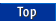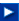Apache C++ Standard Library User's Guide

## 22.7 Non-Member Functions

### 22.7.1 Binary Operators

Class valarray provides a large block of binary operators that allow a program to apply these operators to two valarrays or to a valarray and a T value, where the T can occur on the left- or right-hand side of the expression. As you'd expect, these operators do not modify either of their arguments, but instead return a new valarray containing the result of the operation. Each version that takes two valarray arguments returns the result of applying the operation to the corresponding elements of the arrays. The versions with a T& argument return a valarray whose elements result from applying the operation to each element in the valarray argument along with the T& argument. See the valarray entry in the Apache C++ Standard Library Reference Guide for a complete list of binary operators.

Arithmetic and bitwise operators return a valarray of T. Logical operators return a valarray<bool>, where each element represents the comparison of corresponding elements in two valarrays or the comparison of each element in an array with a particular value. For example:

```int a = {1,2,3};
int b = {0,1,4};
std::valarray<int> v1(a,3);
std::valarray<int> v2(b,3);
std::valarray<int> v3(0,3);
std::valarray<bool> v4(false,3);
v3 = v1 + v2;                      // v3 = {1,3,7}
v4 = v1 == v2;                     // v4 = {false,false,false}
v4 = v1 < v2;                      // v4 = {false,false,true}
```

### 22.7.2 Transcendental Functions

Class valarray also provides 20 transcendental functions, four of which are overloads. Each function returns a new valarray that contains elements resulting from the application of the transcendental function to each element in the valarray passed as an argument. See the valarray entry in the Apache C++ Standard Library Reference Guide for a complete list of transcendental functions.

The following example squares each of the elements of an array:

```using std::pow;

float a = {2.0, 4.0, 16.0};
std::valarray<float> v(a,3);            // v = {2.0, 4.0, 16.0}
std::valarray<float> v2(0.0,3);         // v2 = {0.0, 0.0, 0.0}
v2 = std::pow(v,2.0);                   // v2 = {4.0, 16.0, 256.0 }
```